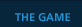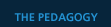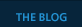по нумерологии число 121 нумерология 9 жизненный путь гороскопу какой нумерология код судьбы человека джули по гороскоп на дева сегодня от павла глобаPHYSICS ABRIDEGED   First and foremost, what we will teach is Physics: Classical, Newtonian, Algebra and Trigonometry based, Conceptual Physics. In line with this Worldview, the Earth is the basis of all our observations. Using a theater model, it sets the stage; it is the stage!On this stage we present the following two sets of actors... KINEMATICS Time (t), Acceleration (a), Velocity (v), Position (x) DYNAMICS Mass (m), Force (F), Momentum (P), Work (W) ...each with it's individual script... KINEMATIC Change in (x) divided by change in (t) is velocity (v) Change in (v) divided by change in (t) is acceleration (a) DYNAMIC A massive object will not have a change in velocity (v) unless acted upon by a net Force (F). Every force (F1) has an opposite twin force (-F2). Acceleration (a) is equal to net Force (F) divided by (m). Velocity (v) is equal to net Momentum (P)) divided by (m) Position (x) is equal to net Work (W) divided by Net Force (F) Conservation Laws: Mass, Energy, Momentum ...and thus the theater of life as Newton knew it is played out. Traditional, Studio, Browser, pAST   Depending on the setting and audience, a Physics class can be conceptual or calculus based. It can cover everything from Newton to Einstein in one semester or focus purely on Mechanics for one year. As our game is aimed at High School and Freshman University students, the scope of our material will be Conceptual Newtonian Mechanics. This means we will cover Kinematics (position, velocity, etc) and Dynamics (force, momentum, etc) with only algebra and trigonometry. A traditional conceptual Physics course is usually broken up into Classical and Modern Physics. Classical encompasses Newtonian Mechanics, Thermodynamics, and Maxwellian Electricity and Magnetism; essentially Physics up until the 20th century. Modern Physics starts with the beginning of the 20th century with Quantum Mechanics and Relativity and then moves on to particle Physics and solid state. The purpose of a conceptual Physics class is therefore to give a wide and shallow presentation of Physics. However it is also used to present a worldview based on the scientific method and Physics laws... an understanding that must be in place before a student tackles any other science or more advanced Physics. To the right we can see a one semester Newtonian Physics syllabus which should give an idea of the flow of material that we should expect in a Traditional course. Physics is traditionally taught using the "teach and preach" method. The professor will lecture for half an hour to an hour and then assign homework. Sometimes as part of the class, sometimes as a different class, the students can also be expected to attend labs for the hands-on experience in exploring the concepts they learn in lecture and homework. Tests and Finals range from multiple choice for conceptual classes to solving lengthy problems for calculus based classes. PER and FCI   In the past 20 years, many questions have been raised as to the decline of retention in science courses. The churn rate in science courses is abnormally high which indicates not a failure of interest but a failure in our ability to focus on our students educational needs and teach effectively. Physics Education Research (PER) has yielded many new and useful arguments on the subject over the last 15 yearsA centerpiece of this effort is the Force Concept Inventory (FCI) test which aims to test conceptual rather than math knowledge. The FCI is a multiple choice test about Physics concepts such as inertia and vectors. It is usually given the first and last days a student is in class and thus provides a pre/post measurement of the students gain in Physics knowledge, known as in the literature. The FCI showed that a student taking a Traditional class had an average 23% gain in Physics knowledge as a result of the class. Interactive Engagement classes however showed double the gain and thus it was quickly shown that classes that emphasized lecture (Traditional) over lab (Interactive) were not adequately transferring knowledge to their students. (web) Computerized and Collaborative pedagogy.